Next: Laplacian Operator Up: Vectors and Vector Fields Previous: Grad Operator

# Divergence

Let us start with a vector field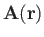. Considerover some closed surface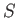, where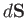denotes an outward pointing surface element. This surface integral is usually called the flux ofout of. Ifrepresents the velocity of some fluid thenis the rate of fluid flow out of.

Ifis constant in space then it is easily demonstrated that the net flux out ofis zero. In fact,(A.129)

because the vector areaof a closed surface is zero.Suppose, now, thatis not uniform in space. Consider a very small rectangular volume over whichhardly varies. The contribution tofrom the two faces normal to the-axis is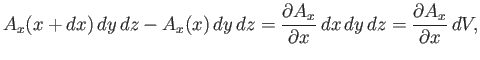(A.130)

whereis the volume element. (See Figure A.21.) There are analogous contributions from the sides normal to the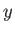- and-axes, so the total of all the contributions is(A.131)

The divergence of a vector field is defined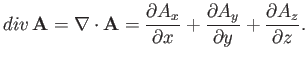(A.132)

Divergence is a good scalar (i.e., it is coordinate independent), because it is the dot product of the vector operatorwith. The formal definition of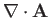is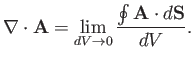(A.133)

This definition is independent of the shape of the infinitesimal volume element.

One of the most important results in vector field theory is the so-called divergence theorem. This states that for any volumesurrounded by a closed surface,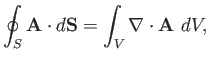(A.134)

whereis an outward pointing volume element. The proof is very straightforward. We divide up the volume into very many infinitesimal cubes, and sumover all of the surfaces. The contributions from the interior surfaces cancel out, leaving just the contribution from the outer surface. (See Figure A.22.) We can use Equation (A.131) for each cube individually. This tells us that the summation is equivalent to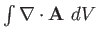over the whole volume. Thus, the integral ofover the outer surface is equal to the integral ofover the whole volume, which proves the divergence theorem.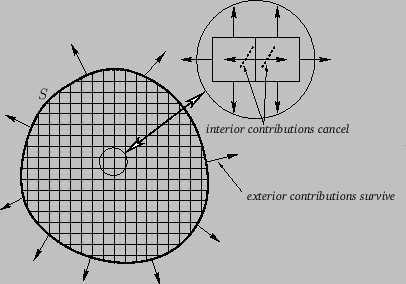Now, for a vector field with,(A.135)

for any closed surface. So, for two surfaces,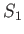and, on the same rim,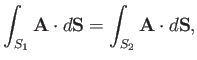(A.136)

as illustrated in Figure A.23. (Note that the direction of the surface elements onhas been reversed relative to those on the closed surface. Hence, the sign of the associated surface integral is also reversed.) Thus, ifthen the surface integral depends on the rim, but not on the nature of the surface that spans it. On the other hand, ifthen the integral depends on both the rim and the surface.Consider an incompressible fluid whose velocity field is. It is clear thatfor any closed surface, because what flows into the surface must flow out again. Thus, according to the divergence theorem,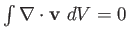for any volume. The only way in which this is possible is ifis everywhere zero. Thus, the velocity components of an incompressible fluid satisfy the following differential relation: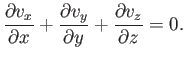(A.137)

It is sometimes helpful to represent a vector fieldby lines of force or field-lines. The direction of a line of force at any point is the same as the local direction of. The density of lines (i.e., the number of lines crossing a unit surface perpendicular to) is equal to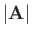. For instance, in Figure A.24,is larger at point 1 than at point 2. The number of lines crossing a surface elementis. So, the net number of lines leaving a closed surface is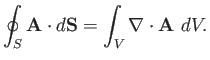(A.138)

Ifthen there is no net flux of lines out of any surface. Such a field is called a solenoidal vector field. The simplest example of a solenoidal vector field is one in which the lines of force all form closed loops.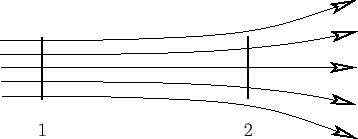Next: Laplacian Operator Up: Vectors and Vector Fields Previous: Grad Operator
Richard Fitzpatrick 2016-03-31